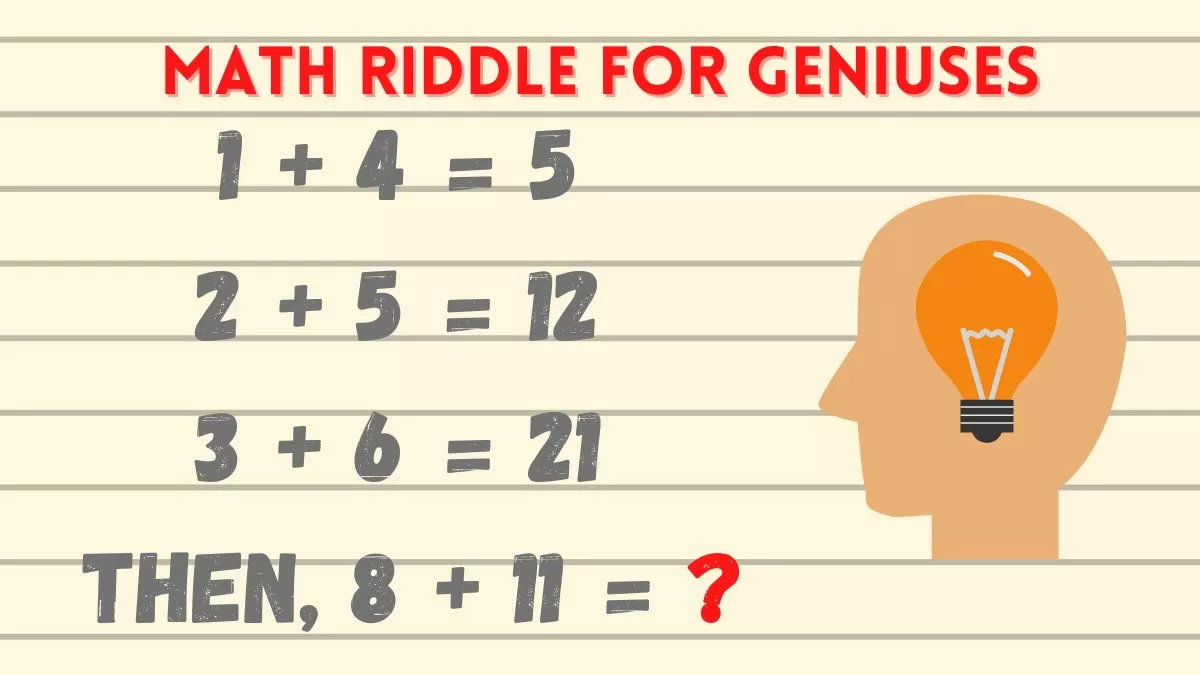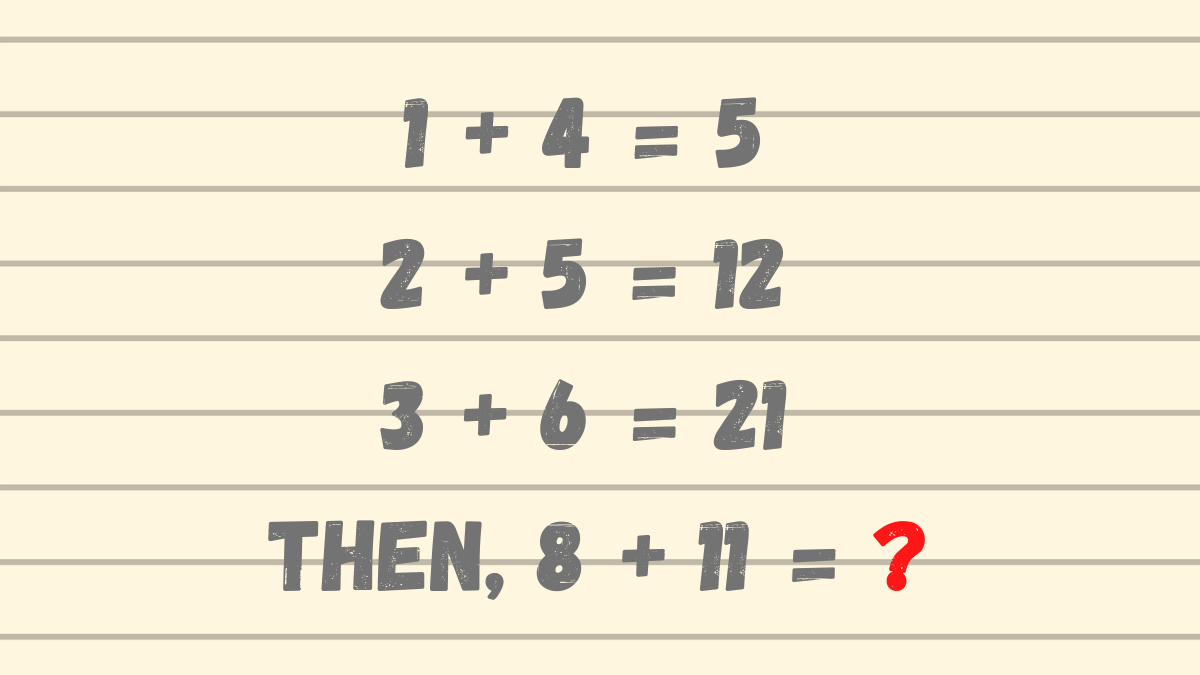This Math Riddle Is Only For Geniuses To Solve. The Majority Will Fail. - Padhle.Online
Students' favourite free learning app with LIVE online classes, instant doubt resolution, unlimited practice for classes 6-12, personalized study app for Maths, Science, Social Studies, video e-learning, online tutorial, and more. Download Now!

# This Math Riddle Is Only For Geniuses To Solve. The Majority Will Fail.This Math Riddle Is Only For Geniuses To Solve. The Majority Will Fail.

Math riddles are great fun and they are also very useful. They allow us to test our knowledge of math concepts such as algebra, geometry, trigonometry, calculus, etc.

However, they do not appeal to all. Only a small percentage of the population likes them. As they need a great deal of critical and analytical thinking and are difficult, intriguing, and thought-provoking.

This math riddle will put your logical thinking and reasoning skills to test.

Are you ready to solve it?

Great! Let’s begin.

# This Math Riddle Is Only For Geniuses To Solve.

Look at the math problem posted below.Can you solve this riddle?

Find this too easy?

Fine, let’s turn this into a challenge.

Set a timer to 11 seconds and try to find the answer within the allotted time.

Good luck!

You must have figured out the answer by now. If you have, congratulations are in order. We knew you could do this.

There are two possible answers to this riddle.

One: 1 + 4 = 5.

Does this logic apply to the next line?

No. 2 + 5 = 7 not 12

Applying a different by adding the previous result to the sum below.

So, 5 + 2 + 5 = 12.

Similarly, 12 + 3 + 6 = 21.

Going by this logic

21 + 8 + 11 = 40.

Hence, 8 + 11 = 40.

However, there is another possible answer to this reasoning riddle.

The numbers on the LHS follow a certain pattern, such as 1 + 4 is followed by the individual numbers’ successors i.e. 2 + 5.

So, what if, 8 + 11 is not the next in the pattern?

What if the pattern is

1 + 4  =   5

2 + 5  = 12

3 + 6  =  21

4 + 7  =  ?

5 + 8  =  ?

6 + 9  =  ?

7 + 10 = ?

8 + 11 =  ?

Following this pattern,

21 + 4 + 7 = 32.

Adding the numbers following the pattern we getThis Math Riddle Is Only For Geniuses To Solve. The Majority Will Fail.

Math riddles are great fun and they are also very useful. They allow us to test our knowledge of math concepts such as algebra, geometry, trigonometry, calculus, etc.

However, they do not appeal to all. Only a small percentage of the population likes them. As they need a great deal of critical and analytical thinking and are difficult, intriguing, and thought-provoking.

This math riddle will put your logical thinking and reasoning skills to test.

Are you ready to solve it?

Great! Let’s begin.

# This Math Riddle Is Only For Geniuses To Solve.

Look at the math problem posted below.Can you solve this riddle?

Find this too easy?

Fine, let’s turn this into a challenge.

Set a timer to 11 seconds and try to find the answer within the allotted time.

Good luck!

You must have figured out the answer by now. If you have, congratulations are in order. We knew you could do this.

There are two possible answers to this riddle.

One: 1 + 4 = 5.

Does this logic apply to the next line?

No. 2 + 5 = 7 not 12

Applying a different by adding the previous result to the sum below.

So, 5 + 2 + 5 = 12.

Similarly, 12 + 3 + 6 = 21.

Going by this logic

21 + 8 + 11 = 40.

Hence, 8 + 11 = 40.

However, there is another possible answer to this reasoning riddle.

The numbers on the LHS follow a certain pattern, such as 1 + 4 is followed by the individual numbers’ successors i.e. 2 + 5.

So, what if, 8 + 11 is not the next in the pattern?

What if the pattern is

1 + 4  =   5

2 + 5  = 12

3 + 6  =  21

4 + 7  =  ?

5 + 8  =  ?

6 + 9  =  ?

7 + 10 = ?

8 + 11 =  ?

Following this pattern,

21 + 4 + 7 = 32.

Adding the numbers following the pattern we get

77 + 8 + 11 = 96.

There is another way to arrive at the 2nd solution.

1 + ( 4 x 1 )

Similarly, 2  + ( 5 x 2 ) = 12

Hence, 8 + ( 11 x 8 ) = 96.

So, there are two possible solutions

40 & 96.

Pretty tough, right?

We hope that you had fun with this math riddle.

both; font-family: Roboto, sans-serif; font-size: 16px; margin: 0px 0px 20px; outline: none; padding: 0px;">77 + 8 + 11 = 96.

There is another way to arrive at the 2nd solution.

1 + ( 4 x 1 )

Similarly, 2  + ( 5 x 2 ) = 12

Hence, 8 + ( 11 x 8 ) = 96.

So, there are two possible solutions

40 & 96.

Pretty tough, right?

We hope that you had fun with this math riddle.

Principal, Babu Daudayal SVM, Mathura